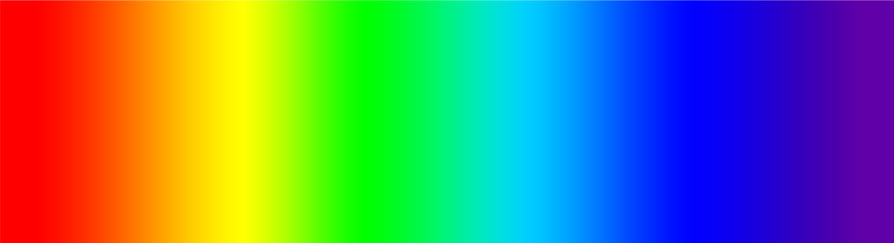# Quantum Phenomena

If light of a high enough frequency is shone onto a metal surface, electrons are emitted which is called the photoelectric effect.

• For a given metal, no photoelectrons are emitted if the incident light has a frequency lower than the threshold frequency of the metal.
• The photoelectrons are emitted with kinetic energy ranging from zero to a maximum, depending on the frequency, though unaffected by the intensity.
• The number of photoelectrons emitted per second is proportional to the intensity.

#### Wave Theory

According to the wave theory:

• If the light has a lower frequency, it should take longer for electrons to be emitted as a small amount of energy would be gained from each wave. This is not the case as electrons are emitted immediately if the frequency is higher than the threshold.

• The higher the intensity, the more energy transferred, and higher the resulting kinetic energy of the photoelectron. This is not the case however, as the kinetic energy depends on the frequency.

#### Photon Model

To counteract these observations, the photon model was developed whereby waves can only be released in discrete energy packets called photons. The energy carried by each of these photons is:

$$E = hf$$

Where $E$ is the energy, $h$ is Plancks constant, and $f$ is the frequency.

According to the photon model, when light hits a metal surface, the metal is bombarded by photons. When one photon collides with one free electron, the electron gains energy equal to $hf$. In order to leave the metal, the electron must gain sufficient energy to overcome the attraction of the atom. This required energy is the work function $\phi$ and is a constant of the metal.

#### Threshold Frequency

To overcome the work function, the incident waves must be over a threshold frequency. This frequency is:

$$f = \frac{\phi}{h}$$

#### Maximum Kinetic Energy

The energy transferred to an electron is $hf$. In order to escape a metal surface, the work function must be exceeded. Any remaining energy is used by the electron as kinetic energy. It is known as the maximum energy as some energy is used in order to leave the surface of the metal.

$$hf = \phi + \frac{1}{~~2}mv_{max}^{2}$$

## Energy Levels

Electrons in atoms exist in discrete energy levels. Each level is given a number with $n = 1$ representing the ground state. Electrons are able to move between these levels through excitation and de-excitation.

#### Excitation

This is where an incident electron collides with an electron in a particular shell. Energy is transferred which allows the electron to transition to a higher state if the collision is of exact energy.

#### De-excitation

This is where an electron falls to a lower energy level, emitting a photon of energy equal to the difference in energy levels. As these transitions are between definite levels, the energy of each photon can only take certain values.

### Electronvolt

The electron volt ($eV$) is defined as the kinetic energy carried by an electron after it has been accelerated through a potential difference of 1 volt.

The energy carried by each photon is equal to the difference in energies between the two levels.

$$hf = E_{2} - E_{1}$$

### Fluorescent Tubes

Fluorescent tubes use the photon emission principle to produce light:

1. The tubes are filled with mercury atoms which become excited due to collisions with fast moving free electrons accelerated by the high voltage.
2. These atoms de-excite, emitting mostly UV radiation.
3. The inside of the tubing is coated with phosphorus which absorbs UV photons, before de-exciting, emitting useful visible light.

## Line Spectra

A line spectra can be produced when light is split using a prism or diffraction grating. On a line spectrum, the series of lines correspond to a particular wavelength of emitted light. As the emitted photons have a discrete wavelength, these lines are caused by the transitions of electrons.### Continuous Spectra

The spectrum of white light is continuous. This means that if the light is split with a prism, the colours produce a full spectrum with no gaps.## Wave Particle Duality

#### Light As a Wave

Light produces interference and diffraction patterns which provide evidence of wave behaviour.

#### Light as a Particle

The photoelectric effect gives evidence for light as a particle as the kinetic energy depends on the frequency of the light not the intensity.

### De Broglie Wavelength

Louis de Broglie came up with the idea that if light showed evidence of particle behaviour, particles should show evidence of wave-like behaviour. The de Broglie equation relates wavelength to momentum:

$$\lambda = \frac {h}{mv}$$

The wave nature of electrons was shown through the production of diffraction patterns, when accelerated electrons in a vacuum interact with the spaces in a graphite crystal.